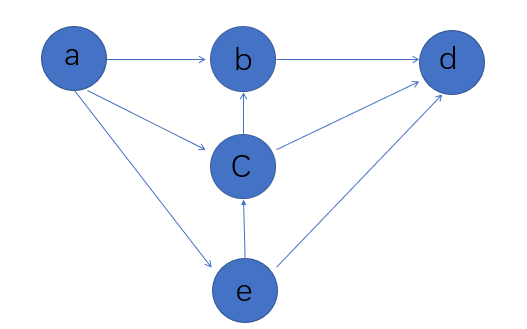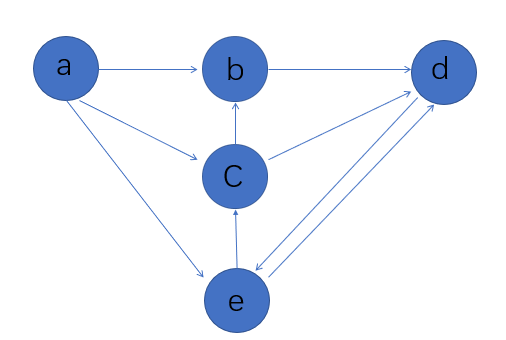# python实现拓扑排序的基本教程

更新时间：2018年03月11日 09:51:50   作者：赵洁钰Amy我要评论

• 每个顶点出现且只出现一次。
• 若A在序列中排在B的前面，则在图中不存在从B到A的路径。

• 从N中选出一个入度为0的顶点作为序列的下一顶点。
• 从N网中删除所选顶点及其所有的出边。
• 反复执行上面两个步骤，知道已经选出了图中的所有顶点，或者再也找不到入度为非0的顶点时算法结束。```def toposort(graph):
in_degrees = dict((u,0) for u in graph) #初始化所有顶点入度为0
vertex_num = len(in_degrees)
for u in graph:
for v in graph[u]:
in_degrees[v] += 1  #计算每个顶点的入度
Q = [u for u in in_degrees if in_degrees[u] == 0] # 筛选入度为0的顶点
Seq = []
while Q:
u = Q.pop()  #默认从最后一个删除
Seq.append(u)
for v in graph[u]:
in_degrees[v] -= 1  #移除其所有指向
if in_degrees[v] == 0:
Q.append(v)   #再次筛选入度为0的顶点
if len(Seq) == vertex_num:  #如果循环结束后存在非0入度的顶点说明图中有环，不存在拓扑排序
return Seq
else:
print("there's a circle.")
G = {
'a':'bce',
'b':'d',
'c':'d',
'd':'',
'e':'cd'
}
print(toposort(G))```

`['a', 'e', 'c', 'b', 'd']``G = { 'a':'bce', 'b':'d','c':'d','d':'e','e':'cd'}`

```there's a circle.
None```